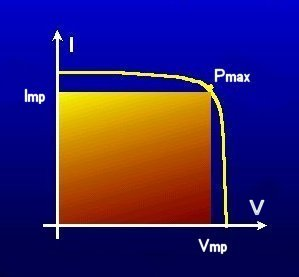A# maximum power pointI-V characteristic of a solar cell, showing maximum power point. Credit: University of Southampton.

The maximum power point (MPP) is the point on the current-voltage (I-V) curve of a solar module under illumination, where the product of current and voltage is maximum (Pmax, measured in watts). The points on the I and V scales which describe this curve point are named Imp (current at maximum power) and Vmp (voltage at maximum power.)

For a typical silicon cell panel, the maximum power point (MPP) is about 17 volts for a 36-cell configuration.